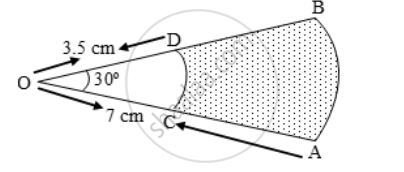# In Fig. there are shown sectors of two concentric circles of radii 7 cm and 3.5 cm. Find the area of the shaded region. - Mathematics

Sum

In Fig. there are shown sectors of two concentric circles of radii 7 cm and 3.5 cm. Find the area of the shaded region. Use π = (\frac { 22 }{ 7 }).

#### Solution

Let A1 and A2 be the areas of sectors OAB and OCD respectively. Then, A1 = Area of a sector of angle 30º in a circle of radius 7 cmA_{1}={ \frac{30}{360}\times \frac{22}7\times 7^{2}}

⇒ A_1 = \frac { 77 }{ 6 } cm^2

A2 = Area of a sector of angle 30º in a circle of radius 3.5 cm.

∴ Area of the shaded region

A_{2}={ \frac{30}{360}\times \frac{22}{7}\times (3.5)^{2}}

A_{2}={ \frac{1}{12}\times \frac{22}{7}\times\frac{7}{2}\times \frac{7}{2}}

=A_{1}-A_{2}=( \frac{77}{6}-\frac{77}{24})

= \frac { 77 }{ 24 } × (4 – 1) cm^2 = \frac { 77 }{ 8 } cm^2 = 9.625 cm^2

Concept: Circumference of a Circle
Is there an error in this question or solution?

Share